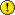Warning

## Toolbox of Elementary and Helper Functions

Following functions should be implemented. It is important to keep the specified calling convention (order of arguments, shape of matrices, etc).

Note: vectors of coordinates (e.g., points) should bealways columns. Thus a set of 5 euclidean points in a plane is written as a matrix with 2 rows and 5 columns (i.e., 2 x 5).

#### e2p — Transformation of euclidean to projective coordinates

 Synopsis u_p = e2p( u_e ) Inputs u_e d by n matrix; n euclidean vectors of dimension d Outputs u_p d+1 by n matrix; n homogeneous vectors of dimension d+1

#### p2e — Transformation of projective to euclidean coordinates

 Synopsis u_e = p2e( u_p ) Inputs u_p d+1 by n matrix; n homogeneous vectors of dimension d+1 Outputs u_e d by n matrix; n euclidean vectors of dimension d
>> u = e2p( [[1;2] [3;4] [5;6]] )

u =
1     3     5
2     4     6
1     1     1

>> p2e( u )

ans =
1     3     5
2     4     6

#### vlen — Column vectors length

 Synopsis l = vlen( x ) Inputs x d by n matrix; n vectors of dimension d Outputs l 1 by n row vector; euclidean lengths of the vectors
>> vlen( [ [0.5;0.5;1] [3;4;0]])

ans =
1.2247    5.0000

#### sqc — Skew-symmetric matrix for cross-product

 Synopsis S = sqc(x) Inputs x vector 3×1 Outputs S skew symmetric matrix (3×3) for cross product with x

Notes: The vector x characterises the left as well as the right null space of the matrix S. For any vector y, cross product x×y equals to S y.

>> sqc( [1;2;3] )

ans =
0    -3     2
3     0    -1
-2     1     0

#### EutoRb — Essential matrix decomposition with cheirality

This is obsolete, create EutoRt instead.

 Synopsis [R, b] = EutoRb( E, u1, u2 ) Inputs E essential matrix (3×3) u1, u2 corresponding image points in homogeneous coordinates (3×n), used for cheirality test Outputs R relative rotation (3×3) or [] if cheirality fails b relative translation, euclidean (3×1), unit length

Notes: The sessential matrix E is decomposed such that E = R * sqc( b ).

#### EutoRt — Essential matrix decomposition with cheirality

 Synopsis [R, t] = EutoRt( E, u1, u2 ) Inputs E essential matrix (3×3) u1, u2 corresponding image points in homogeneous coordinates (3×n), used for cheirality test Outputs R relative rotation (3×3) or [] if cheirality fails t relative translation, euclidean (3×1), unit length

Notes: The sessential matrix E is decomposed such that E ~ sqc(t) * R. Note that t = -R*b.

#### Pu2X — Binocular reconstruction by DLT triangulation

 Synopsis X = Pu2X( P1, P2, u1, u2 ) Inputs P1, P2 projective camera matrices (3×4) u1, u2 corresponding image points in homogeneous coordinates (3×n) Outputs X reconstructed 3D points, homogeneous (4×n)

#### err_F_sampson — Sampson error on epipolar geometry

 Synopsis err = err_F_sampson( F, u1, u2 ) Inputs F fundamental matrix (3×3) u1, u2 corresponding image points in homogeneous coordinates (3×n) Outputs e Squared Sampson error for each correspondence (1×n).

#### u_correct_sampson — Sampson correction of correspondences

 Synopsis [nu1, nu2] = u_correct_sampson( F, u1, u2 ) Inputs F fundamental matrix (3×3) u1, u2 corresponding image points in homogeneous coordinates (3×n) Outputs nu1,nu2 corrected corresponding points, homog. (3×n).